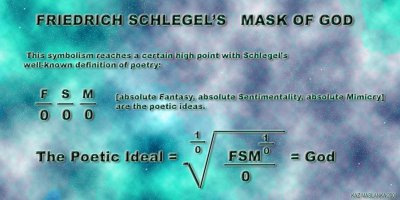## Sunday, August 06, 2006

### Friedrich Schlegel’s Mask of GodMarko Niemi has translated Friedrich Schlegel’s mathematical poem for us. Marko also poses the question what happens if you divide the God by zero one more time.
I would love to hear Paul Gailiunas expound on that question. I think he would shy away from the idea of exploring the idea of God divided by zero but he may give us an answer to what happens when you divide infinity by zero.Anonymous said...

I would think that infinity divided by zero gives us more infinity...

God is often equated to infinity which in turn is often equated to 1 divided by 0....

So, here is one question to twist our brains for a while:

What happens if you multiply God by 0; do you get zero (Oblivion) or 1 (Individual)?

Kaz Maslanka said...

Hi Pablo,
Anything multiplied by zero is zero. I think it is prudent that we follow the rules of math ... in fact we are really bending the rules of math by dividing by zero. It has been my experience that mathematicians cringe when we mathematical poets divide by zero because it is total nonsense. I think they would rather us use the limit as the denominator approaches zero for it is more correct (mathematically). We know that the limit of 1/x as x approaches zero is close to infinity but we also see that math poets like to imply that 1/0 is infinity however that is a poetic judgment not a mathematical one. I personally think that it is important to stick by the mathematical rules or our mathematical poetry will become gibberish and unfortunately much mathematical poetry that is out there on the internet is gibberish for that reason. Infinity divided by zero could be thought of as more infinity however, the mathematical rules state that it is undefined. What is important about this mathematical poem is that it was created around the year 1800 and is the first mathematical poem that I know of. I personally believe it is far better than any of the math poems that I have seen that were created in the 1970's. The main reason I say that is because Schlegal had something to say as opposed to the idea of making math poetry just for the novelty of making it.
Thanks,
Kaz

VizPo-Central said...

Thinking about God divided by infinity (from a hardbound scientific materialist's point of view, I wondered what the word for what I would think of as the opposite of infinity, being the smallest possible number instead of the largest. "Infinitesimal" would be the adjective, I guess, but what would the noun be.

--Bob

Kaz Maslanka said...

-Bob, I think what you are asking for is epsilon.

http://mathworld.wolfram.com/Epsilon.html